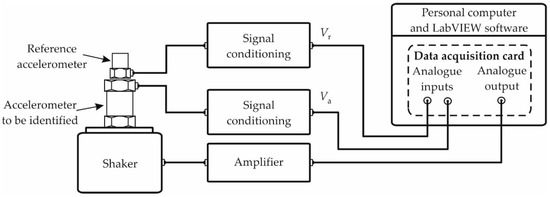Previous Article in Journal
Modeling the Charging Behaviors for Electric Vehicles Based on Ternary Symmetric Kernel Density Estimation
Open AccessArticle

# Monte Carlo-Based Procedure for Determining the Maximum Energy at the Output of Accelerometers

byKrzysztof TomczykFaculty of Electrical and Computer Engineering, Cracow University of Technology, Warszawska 24, 31-155 Krakow, Poland
Energies 2020, 13(7), 1552; https://doi.org/10.3390/en13071552
Received: 13 February 2020 / Revised: 16 March 2020 / Accepted: 26 March 2020 / Published: 27 March 2020
The solutions presented in this paper can be the basis for mutual comparison of different types of accelerometers produced by competing companies. An application of a procedure based on the Monte Carlo method to determine the maximum energy at the output of accelerometers is discussed here. The fixed-point algorithm controlled by the Monte Carlo method is used to determine this energy. This algorithm can only be used for the time-invariant and linear measurement systems. Hence, the accelerometer nonlinearities are not considered here. The mathematical models of the accelerometer and the special filter, represented by the relevant transfer functions, are the basis for the above procedure. Testing results of the voltage-mode accelerometer of type DJB A/1800/V are presented here as an example of an implementation of the solutions proposed. Calculation of the energy was executed in Mathcad 14 program with the built-in Programming Toolbar. The value of the maximum output energy determined for a specified time interval corresponds to the maximum integral-square error of the accelerometer. Such maximum energy can be a comparative ratio just like the accuracy class in the case of instruments used for the static measurements. Hence, the main analytical and technical contributions of this paper concern the development of theoretical procedures and the presentation of their application on the example of a real type of accelerometer. View Full-Text
Show FiguresFigure 1

MDPI and ACS Style

Tomczyk, K. Monte Carlo-Based Procedure for Determining the Maximum Energy at the Output of Accelerometers. Energies 2020, 13, 1552.